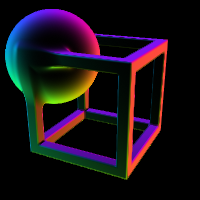# iridescenceContrib

beta
material
iridescenceContrib
RayTK
v0.36

Shading element that produces a rainbow pattern around the edges of shapes, depending on which direction the surface is facing (the surface normal).

## Parameters

• `Enable`:
• `Level`: Overall brightness.
• `Phase`: Shifts the color pattern around the range of surface angles.
• `Period`: How wide the rainbow series is spread across the range of angles. A period of 0.5 will repeat the pattern twice and a period of 2 will only go through half the range before jumping back to the start value.
• `Spread`: The range from the edge where the color is applied, as a 0..1 ratio of how much the surface is facing the camera. The first number is the start of the faded range and the second is the end of it (the place where the color is at full brightness).
• `Enable Shadow`: Whether shadows should be applied to the color.

## Inputs

• `Phase`: (optional) Field that offsets the phase of the color pattern.
• Coordinate Types: `float` `vec2` `vec3` `vec4`
• Context Types: `MaterialContext`
• Return Types: `float`